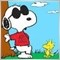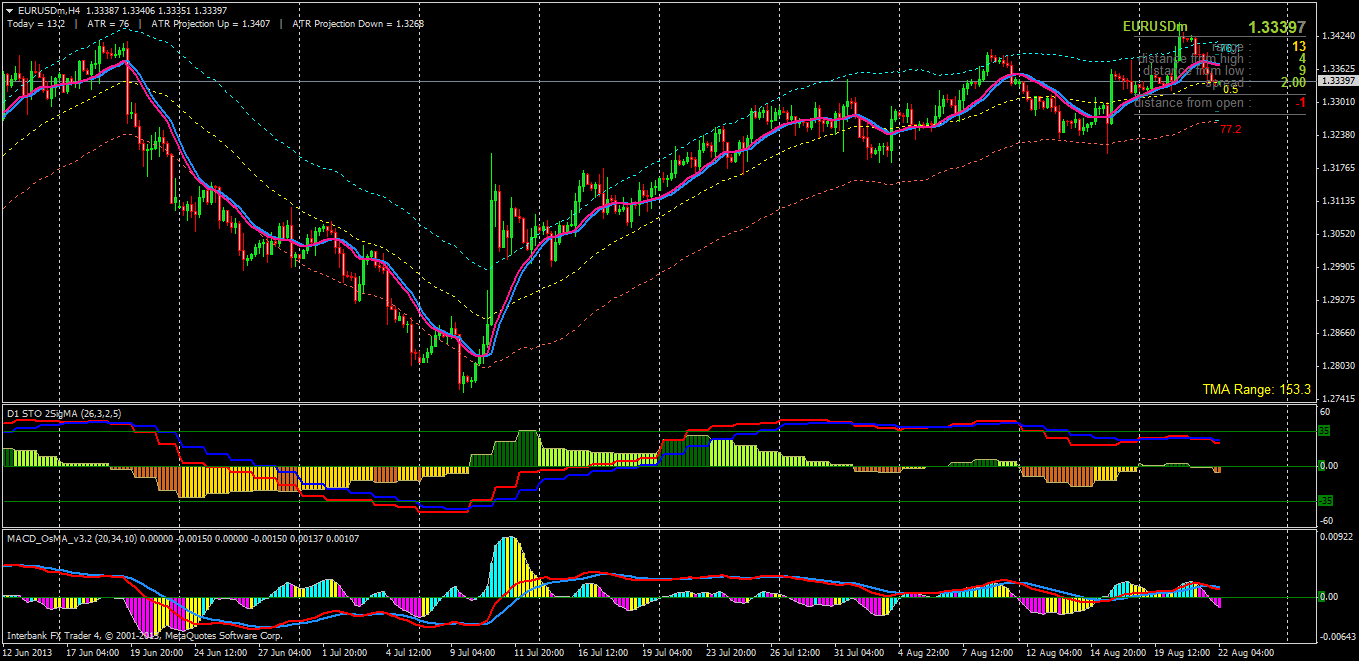# Stochastic Oscillator, the beginning - page 51154433

solograppa:
Hi i need help, i need a stochastic look like this:

Try the one from this link : stochastic_osma_momentum.mq42047

AllStochastic V4

Files:9492

This is stochastic adaptive smoother indicator with alerts and arrows.The picture is showing default settings.

Files:200

This stochastic with 2 sigma lines and histogram is "multi-time frame" (originally introduced by mladen and changed by mrtools). In the last month I discovered the real value of this multi-time frame function. It is a wonderful filter for the finding the best trades. I trade the 4hr TF and set the indicator to D1. I generally trade in the direction of trends and only trade counter trends/reversals that agree with this indicator. Picture is attached for reference. BTW, you only see two lines because I only show the two sigmas and not the actual stochastic...by setting the color of the stochastic to none.stochastic_2sigmas_mtfhisto.mq4

Files:
euraudmh4.png 68 kb36

gcgman,8

mrtools:
This is stochastic adaptive smoother indicator with alerts and arrows.The picture is showing default settings.

Sorry, i know. Thanks for indicator!154433

There are some stochastic indicators of rsi already. This one differs because it is using a "classical" stochastic calculation for that instead of "raw" stochastic. It is all a part of an experiment with a function that calculates stochastic and can take any values as arguments for calculating it. At a first glance the "classical" stochastic of a rsi looks useful too

_________________

PS: on the example upper is the rsi and lower is the stochastic of that same rsi as a comparison what effect can you expect of calculating stochastic of some value

Files:154433

Having a stochastic calculation that can use custom prices for calculation allows us to filter those prices prior to using them in calculation. That way we are not smoothing the already calculated stochastic nor we are calculating a "raw" stochastic of some filtered value but we are "intervening" in the core of the stochastic calculation and we can make a new kind of stochastic that way

______________

This one is a sort of it. If you set the filter to <= 1 then you are going to get a good old high-low stochastic, otherwise close, high and low are going to first be filtered (using super smoother in this case) and only then used in stochastic calculation. Result is a smoother stochastic with minimal lag added

Files:57

There are some stochastic indicators of rsi already. This one differs because it is using a "classical" stochastic calculation for that instead of "raw" stochastic. It is all a part of an experiment with a function that calculates stochastic and can take any values as arguments for calculating it. At a first glance the "classical" stochastic of a rsi looks useful too

_________________

PS: on the example upper is the rsi and lower is the stochastic of that same rsi as a comparison what effect can you expect of calculating stochastic of some value

Thanks for your sharing. Closely watching behavior of this Stoch-of-rsi (SoR) in relation to original RSI, I think that SoR could be seen as volatility measure of related RSI. If this is true, we can take this SoR value to make RSI smoother and/or more adaptive in some way, maybe as alpha factor in smoothing MA or deviation adaptive mode. I don't know if there is any trial before and its result. If not yet, could you please give it a hand Mladen !

Regards,

fareastol154433

fareastol:

Thanks for your sharing. Closely watching behavior of this Stoch-of-rsi (SoR) in relation to original RSI, I think that SoR could be seen as volatility measure of related RSI. If this is true, we can take this SoR value to make RSI smoother and/or more adaptive in some way, maybe as alpha factor in smoothing MA or deviation adaptive mode. I don't know if there is any trial before and its result. If not yet, could you please give it a hand Mladen !